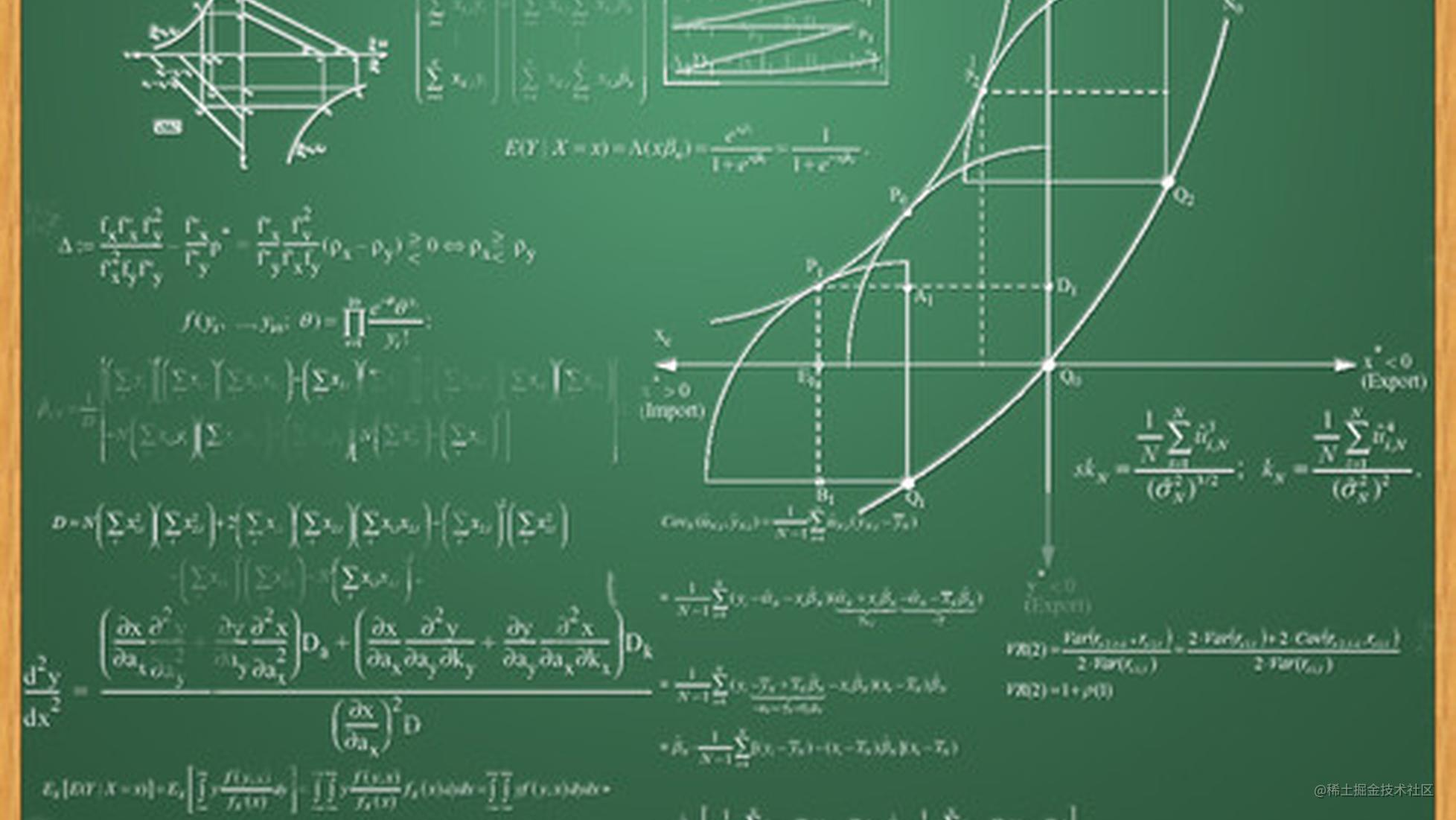# 算法之美：栈和队列

### 由两个栈实现一个队列 （✭✭✩✩✩）

1. 无论如果 StackA（最开始add元素的那个栈） 要往 StackB 中压入元素，那么必须选择一次性全部压入。
2. 无论什么时候从队列中取元素，必须保证元素是从 StackB 中 pop 出的，也就是说，当 StackB 不为空的时候绝不能再次向 StackB 中压入元素。

``````    public static class  TwoStackQueue<E>{
private Stack<E> stackA;
private Stack<E> stackB;

public TwoStackQueue() {
stackA = new Stack<>();
stackB = new Stack<>();
}

/**
* 添加元素逻辑
* @param e 要添加的元素
* @return 这里只是遵循 Queue 的习惯，这里简单处理返回 true 即可
*/
stackA.push(e);
return true;
}

/**
* 去除元素的时候需要判断两个地方，StackA & StackB 是否都为空
* StackB 为空的时候讲StackA中的元素全部依次压入 StackB
* @return 返回队列中的元素 如果队列为空返回 null
*/
public E poll(){
//如果队列中没有元素则直接返回空，也可以选择抛出异常
if (stackB.isEmpty() && stackA.isEmpty()){
return null;
}

if (stackB.isEmpty()){
while (!stackA.isEmpty()){
}
}

return stackB.pop();
}

/**
* peek 操作不取出元素，只返回队列头部的元素值
* @return 队列头部的元素值
*/
public E peek(){
//如果队列中没有元素则直接返回空，也可以选择抛出异常
if (stackB.isEmpty() && stackA.isEmpty()){
return null;
}

if (stackB.isEmpty()){
while (!stackA.isEmpty()){
}
}

return stackB.peek();
}
}

``````#include <stdio.h>
#include <stack>
using namespace std;

template <typename T> class TStackQueue
{
public:
T poll();

private:
stack<T> stackA;
stack<T> stackB;
};

template <typename T> void TStackQueue<T>::add(T node) {
stackA.push(node);
}
template<typename T> T TStackQueue<T>::poll(){
if (stackB.empty() && stackA.empty()) {
return NULL;
}

if (stackB.empty()) {
while (!stackA.empty()) {
stackB.push(stackA.top());
stackA.pop();
}
}
T node = stackB.top();
stackB.pop();
return node;
}

### 两个队列实现一个栈 （✭✭✩✩✩）

``````//如果 queueA 的大小不为 0 则循环取出元素
while(queueA.size() > 0){
//被取出的元素
int result = queueA.poll();
// 这里注意我们取出元素后再去判断一次，队列是否为空，如果为空代表是最后一个元素
if(queueA.size() != 0){
}else{
return result;
}
}

1. 任何时候两个队列总有一个是空的。
3. 取出元素的时候总是将元素除队尾最后一个元素外，导入另一空队列中，最后一个元素出队。

``````public static class TwoQueueStack<E> {
private Queue<E> queueA;
private Queue<E> queueB;

public TwoQueueStack() {
}

/**
* 选一个非空的队列入队
*
* @param e
* @return
*/
public E push(E e) {
if (queueA.size() != 0) {
System.out.println("从 queueA 入队 " + e);
} else if (queueB.size() != 0) {
System.out.println("从 queueB 入队 " + e);
} else {
System.out.println("从 queueA 入队 " + e);
}
return e;
}

public E pop() {
if (queueA.size() == 0 && queueB.size() == 0) {
return null;
}

E result = null;
if (queueA.size() != 0) {
while (queueA.size() > 0) {
result = queueA.poll();
if (queueA.size() != 0) {
System.out.println("从 queueA 出队 并 queueB 入队 " + result);
}
}
System.out.println("从 queueA 出队 " + result);

} else {
while (queueB.size() > 0) {
result = queueB.poll();
if (queueB.size() != 0) {
System.out.println("从 queueB 出队 并 queueA 入队 " + result);
}
}
System.out.println("从 queueB 出队" + result);
}
return result;
}
}

`````` public static void main(String[] args) {
TwoQueueStack<Integer> queueStack = new TwoQueueStack<>();
queueStack.push(1);
queueStack.push(2);
queueStack.push(3);
queueStack.push(4);
queueStack.pop();
queueStack.pop();
queueStack.push(5);
queueStack.pop();
}

``````从 queueA 入队 1

``````#include <stdio.h>
#include<queue>
#include<exception>

using namespace std;

template <typename T> class TQueueStack
{
public:
void push(const T& node);
T pop();

private:
queue<T> queueA;
queue<T> queueB;
};

// 插入元素
template<typename T> void TQueueStack<T>::push(const T& node)
{

//插入到非空队列，如果均为空则插入到queueB中
if (queueA.size() == 0)
{
queueB.push(node);
}
else
{
queueA.push(node);
}
}

template<typename T> T TQueueStack<T>::pop()
{
if (queueA.size() == 0 && queueB.size() == 0)
{
return NULL;
}
if (queueA.size() > 0)
{
while (queueA.size()>1)
{
//queueA中的元素依次删除，并插入到queueB中，其中queueA删除最后一个元素
//相当于从栈中弹出队尾元素
T& data = queueA.front();
queueA.pop();
queueB.push(data);
}
queueA.pop();
}
else
{
while (queueB.size()>1)
{
//queueB 中的元素依次删除，并插入到 queueA 中，其中 queueB 删除最后一个元素
//相当于从栈中弹出队尾元素

T& data = queueB.front();
queueB.pop();
queueA.push(data);
}
queueB.pop();
}
}

### 判断出栈顺序是否符合要求（✭✭✭✩✩）

1. 执行放入操作后，如果栈顶的元素等于对应角标在 popA 数组中的元素值，那么就需要出栈该元素，同事角标加1
2. 如果栈顶的元素不等于对应角标在 popA 数组中的元素值，那么就执行放入操作
3. 待所有的元素都被放入栈中，此时如果栈为空，那么 popA 就是一个出栈序列，反之则不是。

``````public static class Solution {

public boolean IsPopOrder(int[] pushA, int[] popA) {
int len = pushA.length;

Stack<Integer> stack = new Stack<>();
for (int pushIndex = 0, popIndex = 0; pushIndex < len; pushIndex++) {
stack.push(pushA[pushIndex]);
//如果栈顶元素等于 popA[popIndex] 则一直出栈且 popIndex++
while (popIndex < popA.length && popA[popIndex] == stack.peek()) {
stack.pop();
popIndex++;
}
}
return stack.isEmpty();
}
}

C++实现如下

``````class Solution {
public:
bool IsPopOrder(vector<int> pushA, vector<int> popA) {
if(pushA() == 0) return false;
vector<int> stack;
for(int i = 0,j = 0 ;i < pushA.size();){
stack.push_back(pushA[i++]);
while(j < popA.size() && stack.back() == popA[j]){
stack.pop_back();
j++;
}
}
return stack.empty();
}
};

``````public static void main(String[] args) {

Solution solution = new Solution();
int[] pushA = new int[]{1, 2, 3, 4, 5};
int[] popA1 = new int[]{4, 3, 5, 1, 2};
int[] popA2 = new int[]{4, 5, 3, 2, 1};

System.out.println("popA1 是否是出栈队列 " + solution.IsPopOrder(pushA, popA1));
System.out.println("popA2 是否是出栈队列 " + solution.IsPopOrder(pushA, popA2));
}
// 结果
//popA1 是否是出栈队列 false
//popA2 是否是出栈队列 true